# Clocks

Clocks” is one of the prime topics one should study to solve the questions appearing in the public sector entrance exams. It is a significant part of logical reasoning, which not only involves an in-depth logical analysis but also pertains to a detailed level of calculation to solve the questions in the right manner.

In this article, we bring to you the detailed concept of Clocks and the types of questions which may be asked regarding the same. Also, candidates can practise the same clock questions PDF given in this article to get a better understanding of the topic. It is a common topic with regard to the various Government exams conducted across the country.

 Score more in the Reasoning Ability and General Intelligence Section with the help of links given below:

Every year, Clocks form an integral part of the question paper of the following list of Govt. exams that include:

1. IBPS Specialist Officer
2. RRB Assistant Loco Pilot
3. Staff Selection Commission (SSC)
4. SBI PO
5. SBI Clerk
6. SBI SO
7. IBPS PO
8. IBPS Clerk

To explore various SBI exams, check at the linked article.

Check out the video given below to understand the concept of Clocks even better and analyse the best ways to answer questions under this section:## Introduction

A Clock is a circular device provided with three hands viz. an hour hand, minute and second hand. The study of the clock is known as “horology”.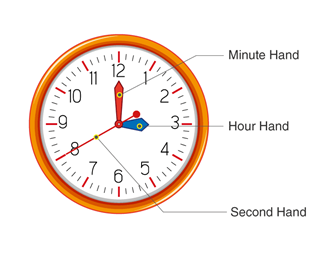A basic structure of a clock with three hands

### Structure of a Clock

A clock is composed of 360 degrees and divided into 12 equal divisions. The angle between the consecutive divisions is obtained by dividing the total angle of clock 360° by the number of divisions i.e. 12.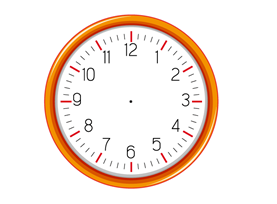Twelve equal divisions of a clock

The angle between any two consecutive divisions = (360°)/12= 30°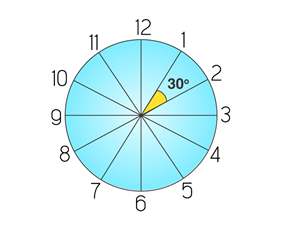Angle divisions of a clock

A close observation of a clock reveals that an angular space between any two consecutive divisions has further five more divisions. The area between the two divisions corresponds to a value of 5 minutes. Hence, dividing the 30° by five will result in the angular value of a minute.

Angular value of a minute = (30°)/5= 6°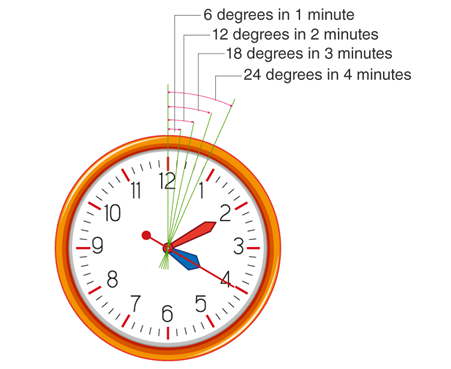Angular values of minutes

To know more about SBI PO Syllabus, check at the linked article.

Given below are a few reasoning ability concepts to prepare for the upcoming Government exams:

### Angle equivalence of a minute

The table given below demonstrates the angular values of the first ten minutes:

 Minute(s) Angular values 1 6° 2 12° 3 18° 4 24° 5 30° 6 36° 7 42° 8 48° 9 54° 10 60°

### Speed of the hands

A clock has three hooks and all three move at different rates. The speed of moving object depends on the distance travelled and the time taken to cover a specific range.

The speed is calculated by:

Speed = Distance/(Time taken)

The speed of a minute hand:

A minute hand travels 360° in one hour. i.e. it travels through all the 12 divisions around the clock every hour. (1 hour = 60 minutes)

Speed of a minute hand = (360°)/(60 minutes)

Speed of a minute hand = 6° per minute.

The speed of an hour hand:

An hour hand travels 30° in an hour. i.e. it covers a distance of 5 minutes (the gap between consecutive divisions) in 60 minutes.

Speed of an hour hand = (30°)/(60 minutes)

Speed of an hour hand =1/2 ° per minute.

Aspirants can check the detailed section-wise syllabus for the various Government exams at the articles linked below:

### Comparison of Speed of hands

The difference in the speed = 6°– (1/2°) = 5.5° per minute

Comparing the speed of the minute hand and an hour hand, one can conclude that the minute hand is always faster than the hour hand by 5.5° or an hour hand is always slower than the minute hand by 5.5°

Note: The evaluation of the speed of second hands is not necessary as it travels a corresponding distance of 1 second in a second.

Frequency of coincidence and collision of hands of a clock:

As we know the hands of clock moves at different speeds, they coincide and collide and also make different angle formations among themselves at various times in a day.

Q.1 How many times in a day do the minute and hour hands of a clock coincide (Angle between them is zero) with each other?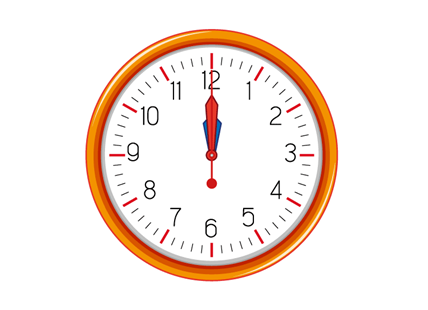A first collision of the hands in a clock at midnight

Logical explanation:

A day starts at midnight, and hence the first-ever coinciding of hands happen at midnight. Now observing the clock, the next coincidence will occur at approximately 1’ o’clock and 5 minutes. Thus, one can conclude that every hour, there is one coincidence of hands. Therefore, the answer should be 24 times for 24 hours. But it is not the correct answer and right logic.

Now observe the time between 11 to 12, either it can either be A.M or P.M, the hands are not coinciding between 11’o o’clock and 12’o o’clock. The coinciding of hands at 12’ o clock is the coincidence between 12 and 1 and 11 and 12. Hence, in 12 hours, there will only be 11 coincidence, extending the logic for 24 hours of the day, there will be 22 coincidences.

If 12:0:0 A.M is the first coincidence of the hands in a day then the next collision will be at 1’o o’clock 5 minutes, but the evaluation of seconds is difficult, but not impossible.

Logical calculation:

We know in 12 hours there will be 11 coincidences. Therefore, one collision will happen at:

Frequency of one collision = (12 hours)/11

Frequency of one collision = (12*60 mins)/11

Frequency of one collision = (720 mins)/11

Frequency of one collision = 65(5/11)

The value 65(5/11) indicates that the hands of a clock coincide after every 65 minutes 5/11 of a minute. i.e. if 12:0:0 is the first collision, then the exact time of the next collision will be obtained by adding 65(5/11) to 12 o’clock.

The below table denotes the time at which both the hands of a clock collide:

 Frequency of collision Time in mixed fraction Exact time 1st 12:0:0 12:0:0 2nd 1:5:5/11 1:5:27 3rd 2:10:10/11 2:10:54 4th 3:16:16/11 3:16:21 5th 4:21:9/11 4:21:16 6th 5:27:3/11 5:27:36 7th 6:32:8/11 6:32:43 8th 7:38:2/11 7:38:10 9th 8:43:7/11 8:43:38 10th 9:49:1/11 9:49:5 11th 10:54:6/11 10:54:32 12th 11:59:11/11 12:0:0

Q.2 How many times in a day do the minute and hour hands of clock form a 180° straight line in a day?

The hands of the clock make one 180° straight line every hour except between 5’o clock and 6’o clock. A precise observation and analysis of the watch gives the idea that between 5 and 6 the hands make straight line 180° exactly at 6 o’clock and hence, it cannot be the one which happens between 5 o’clock and 6 o’clock. Instead it is considered as a straight line formed between 6 and 7 o’clock.

Therefore, the hands of a clock make 180° straight lines 11 times in 12 hours and hence generalising it for 24 hours, the hands make 22 consecutive lines of 180° in 24 hours.

To explore IBPS RRB Syllabus, check at the linked article.

Finding the time when the angle is known:

When the angle between the hands are not perfect angles like 180°, 90° or 270°, the solving of the questions becomes difficult and time-consuming at the same time. The logic below provides a trick to address problems involving angles of hands for other than standard aspects.

T = 2/11 [H*30±A]

Where:

1. T stands for the time at which the angle formed.
2. H stands for an hour, which is running.

(If the question is for the duration between 4 o’clock and 5 o’clock, it’s the 4th hour which is running hence the value of H will be ‘4’.)

1. A stands for the angle at which the hands are at present.

(The value of A is provided in the question generally)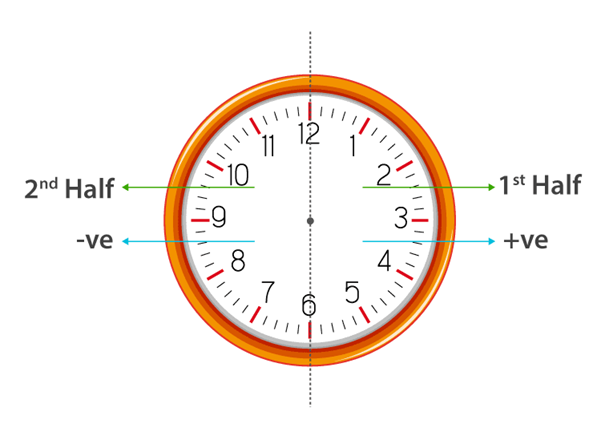The clock is divided into two parts: 1st and 2nd half as shown above

If the time given in the question lies in the first half, then the positive sign is considered while evaluating the time else, then the negative sign is used.

Q3: At what time between 3 and 4 o’clock, the hands makes an angle of 10 degrees?

Solution:

Given: H = 3 , A = 10

Since both three and four lies in the first half considered a positive sign.

Calculations:

T = 2/11 [H*30±A]

T = 2/11 [3*30+10]

T = 2/11 [90+10]

T = 2/11 

T = 200/11

T =18 2/11

The answer indicates that the hands of a clock will make an angle of 10 between 3 and 4 o’clock at exactly 3:18:2/11 ( 3’ o clock 18 minutes and 2/11 of minutes = 2/11*60 = 10.9 seconds)

A few other links to logical reasoning based concept have been given below in the table, candidates can refer to the these for any kind of assistance:

Correct clock v/s Wrong clock:

This section involves the comparison of time in the accurate clock with the wrong watch. The wrong time indicates that a clock is either slow or fast compared to the correct time. The wrong clock can either be fast or delayed by a few seconds/minutes/ hours or sometimes by a few days and weeks.

Q.4 A clock gains 5 seconds for every 3 minutes. If the clock started working at 7 a.m. in the morning, then what will be the time in the wrong clock at 4 p.m. on the same day?

Solution:

A clock gains 5 seconds for every 3 minutes, then it will gain 50 seconds in 30 minutes, or it will acquire 100 seconds in 60 minutes. i.e. it will gain 100 seconds in 1 hour. Since the clock was started at 7 a.m. in the morning and right now the correct time is 4 p.m. the total time the clock has worked is 9 hours. We know that in 1 hour it gains 100 seconds then in 9 hours it increases 900 seconds. The conversion of 900 seconds to minutes will be 15 minutes. This increase indicates that a clock is faster by 15 minutes as the clock is gaining. Hence, the time in the watch would be 4:15 p.m.

To know more about SSC JE Syllabus, check at the linked article.

Puzzle:

Observe the monument. How many minute and hour hand/hands does Big Ben tower clock have?Answer: It has 4-minute hands and 4-hour hands as there are four clocks on all the four sides of it.

Solved examples:

1. An accurate clock shows 7 a.m. Through how many degrees will the hour hand rotate when the clock shows 1 p.m.?

A. 154° B. 180°

C. 170° D. 160°

Solution:

We know that angle traced by hour hand in 12 hrs. = 360°

From 7 to 1, there are 6 hours.

Angle traced by the hour hand in 6 hours =6*(360/12)=180°

Option B is the correct answer.

2. By 20 minutes past 4, the hour hand has turned through how many degrees? If then the clock is 12 p.m.

A. 100° B. 110°

C. 120° D. 130°

Solution:

At 4 o’clock the hour hand is at 4 and has an angle of 30°*4=120°

An Hour hand travels 1/2° per minute In 20 minutes it will travel 20 *(1/2°) = 10°. Adding both we get 120° + 10° = 130°

Option D is the correct answer.

3. At what time between 5.30 and 6 will the hands of a clock be at right angles?

A. 44 minutes past 5 B.44 ( 7/11) minutes past 5

C.43 ( 7/11) minutes past 5 D. 43 minutes past 5

Solution:

Given: H = 5 and A = 90, since 5 and 6 lies in the first half, a positive sign is considered.

T = 2/11 [H*30±A]

T = 2/11 [5*30+90]

T = 2/11  = 480/11= 43( 7/11)

Option C is the correct answer.

4. What is the angle between the minute hand and the hour hand of a clock at 5.30?

A. 05° B. 15°

C. 25° D. 35°

Solution:

At 5 ‘o’clock the hour hand is at 5 and hence has made 30° angle.

From 5 to 5.30 its will travel for 30 minutes with a speed of ½ ° Therefore the total distance travelled will be 30 minutes* 1/2 = 15°

The full angle made by the hour hand will be 150°+15° = 165°.

The minute hand at 5 o’clock is at 12, and hence the angle made is zero. In 30 minutes, it will travel a distance of 30 minutes with a speed of 6° per minute. Therefore, the total distance travelled will be 30 minutes*6° = 180 °.

The angle between the minute and hour hand is 180 – 165 = 15

Option B is the correct answer.

To know more about RRB ALP Syllabus, check at the linked article.

5. How many times in a day, the hands of a clock are straight?

A. 22 B. 24

C. 44 D. 48

Solution:

The hands of clocks make a straight line of 180° about 22 times in 24 hours. Also, the hands coincide 22 times in 24 hours, the coincidence of the hands also forms a straight line. Hence, the total straight lines are 22+22 = 44.

Option C is the correct answer.

6. A house has two wall clocks, one in kitchen and one more in the bedroom. The time displayed on both the watches is 12.A.M right now. The clock in the bedroom gains five minutes every hour, whereas the one in the kitchen is slower by five minutes every hour. When will both the watches show the same time again?

Solution:

The faster clock runs 5 minutes faster in 1 hr.

The slower clock runs 5 minutes slower in 1 hr.

Therefore, in 1 hour, the faster clock will trace 5+5=10 min more when compared to the slower clock.

The table given below depicts the time difference between the slower and faster clock:

 Correct time Slower clock Faster clock 12:0:0 12:0:0 12:0:0 1:0:0 12:55:0 1:5:0 2:0:0 1:50:0 2:10:0 3:0:0 2:45:0 3:15:0 4:0:0 3:40:0 4:20:0 5:0:0 4:35:0 5:25:0 6:0:0 5:30:0 6:30:0

In 6 hours, the faster clock will trace 10×6=60 minutes (an hour) more when compared to the slower clock.

In 6×12=72 hours, the faster clock will trace an hour more when compared to the slower clock since the quicker clock determines 12 hours more than, the slower clock. At this point, both the clocks will show the same time. i.e., both the clocks will show the same time after exactly 72 hours (or 3 days).

Each year the level of Government exam intensifies and with minimum vacancies, the number of aspirants is very high to compete with. Thus, to ace the examinations it is important that candidates leave no stone underturned and ensure that every topic under each section is covered and well prepared.

Also, if candidates are facing any trouble with any of the logical reasoning based concepts, they can turn to BYJU’S for assistance. Not just that, also to get the latest exam pattern, study material, preparation tips or any other exam related information, candidates can turn to the same source.